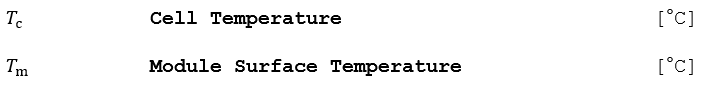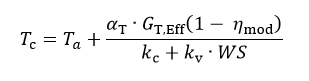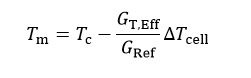# Heat Balance Temperature Model

The thermal behavior of the field, which strongly influences the electrical performances, is determined by a thermal balance between the ambient temperature and the cell’s temperature, which is elevated by incident irradiation. In this simple model, αT is the absorption coefficient of solar irradiation, and ηm is the PV efficiency (related to the Module area), i.e. the removed energy from the module.

## Inputs## Outputs## Algorithm

1.) Compute the module temperature as a function of the available insolation, wind speed, and air temperature:The usual value of the absorption coefficient α is 0.9. The PV efficiency is taken from the Module File.

The thermal behavior is characterized by a thermal loss factor designated here by k, which can be split into a constant component kc and a factor proportional to the wind velocity kv. These factors depend on the mounting mode of the modules (sheds, roofing, facade, etc.).

2.) Convert the cell temperature to a module surface temperature.## Reference

Mermoud, A., Conception et dimensionnement de systèmes photovoltaïques : Introduction des modules PV en couches minces dans le Logiciel PVsyst. Université de Genève, 2005.